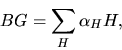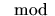Next: Joseph Neisendorfer - James-Hopf Up: 2)  Homotopy Theory / Théorie Previous: L. Gaunce Lewis, Jr.

## John Martino - A Minami-Webb formula for compact Lie groups

 JOHN MARTINO, Western Michigan University, Kalamazoo, Michigan  49008, USA A Minami-Webb formula for compact Lie groups

The Minami-Webb formula expresses the classifying space of a finite group G as the linear combination,of-p cyclic groups (i.e., extensions of p-groups by cyclic p'-groups). The formula and its variants have proven very useful in calculating group cohomology. We generalize the Minami-Webb formula to compact Lie groups.